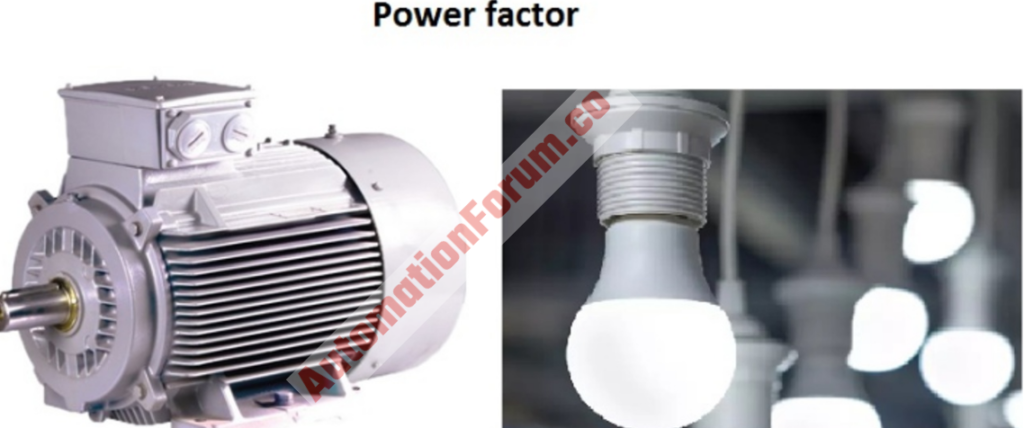# What is the power factor? What is the use of the power factor?The power factor can be described as the relation between the working power and reactive power. It is the measure of how properly we consume our electric power. If the power factor is low then it could cause poor voltage regulation, and the KVA rating of the machine would be high it can also decrease the life of switchgear, motor, etc. So we must maintain a proper power factor and it can be done by power factor correction.

The inductive loads will need a magnetic field but it won’t do any work, so in an AC circuit we would need two types of power components in order to meet the load requirements and these two power components are active and reactive power. The power factor is the measure of the power that is effectively converted into useful power.

#### How to calculate the power factor?

Power factor = Active power/ Total power = P/  S   = KW/ KVA

P= power consumed in the load

S= Total power

So the power factor is the measure of how electric power is utilized properly for the conversion of current to work. If the power factor is high then the power is utilized effectively.

#### What is reactive power?

Reactive power can be considered as a non-working power and reactive power is caused by magnetizing. The apparent power is increased by the reactive power that is required for the inductive loads in a distribution system. This increase in reactive and apparent power will reduce the power factor.

#### How can we improve the power factor?

The power factor can be improved by providing K V A R to the inductive type loads and to provide the K V A R’s we can use a capacitor. So we can improve the power factor of the connected loads by installing power factor improvement capacitors/ capacitor banks. We can improve the power factor by using correctors, synchronous generators, and synchronous motors. It can also be improved by reducing the operation of idling or lightly loaded motors, replace standard motors as they burn out with energy-efficient motors, and we must not operate equipment above its rated voltage.

• It can be improved by using thyristor controlled devices
• Use of DC-DC converters

#### What are the advantages of a good power factor?

• Reduction of losses
• Reduction of voltage drop
• Electrical lines can be utilized better
• Electrical machines can be utilized properly
• The improved voltage will be good to increase the life of the transformer, cables, switchgear, motor, etc.
• The electricity bill will be reduced
• Power consumption will be reduced due to the efficiency and because of this less greenhouse gas emission and fossil fuel depletion by the power station.

#### What are the real power and apparent power?

Real power can be described as the ability of the circuit to perform work at a particular time, while apparent power is the product of current and voltage in the circuit.

#### What are the causes of the low power factor?

The low power factor is caused by inductive loads because they are the sources of reactive power. Some examples of inductive loads are transformer, motor, etc.

#### How power factor correction is done?

Power factor correction is the process to improve the power factor

Static power factor correction

This type of power factor correction uses correction capacitors, the correction capacitors can be connected to the motor starters.

Bulk power factor correction

In this type the total current supplied to the distribution board is monitored by a power factor controller and this device would switch the capacitor banks in a way to maintain the power factor. This bulk correction must not be applied to a lightly loaded transformer. If we do this to an unloaded transformer, then we would create a high resonant circuit between the leakage reactance of the transformer and the capacitors and this would create high voltages.

#### What are the disadvantages of the low power factor?

• The conductor size will be bigger
• Large KVA ratings of the machine
• Large copper losses
• Poor voltage regulation
• The reduced handling capacity of the system

Scroll to Top